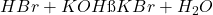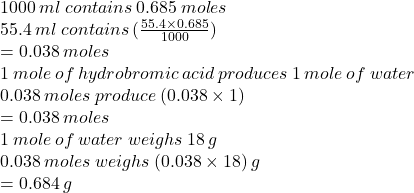Question

Assume that the reaction of aqueous hydrobromic acid solution and potassium hydroxide base undergoes a complete neutralization reaction.

a. Write a balanced chemical equation.

b. How many grams of water can be produce from the complete reaction of excess hydrobromic acid and 55.4 mL of 0.685 M potassium hydroxide solution, assuming that potassium hydroxide is the limiting reactant?

A solution of hydrobromic acid is formed by dissolving 5.00 grams in enough water to make 1.5 L solution.

c.. What was the molarity of this solution?

d. What is the pH of this solution?

1.minhkhue

a.b.c.

0.042 M

d.

1.4